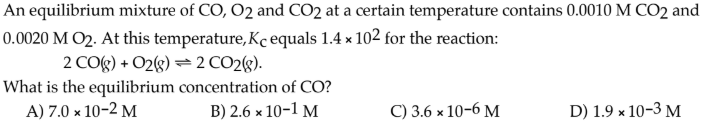# An equilibrium mixture of CO, O2 and CO2 at a certain temperature contains 0.0010 M CO2 and 0.0020 M O2. At this temperature, Kc equals 1.4 × 10^2 for the reaction: 2 CO(g) + O2(g) ⇌ 2 CO2(g). What is the equilibrium concentration of CO? A) 7.0 × 10^-2 M B) 2.6 × 10^-1 M C) 3.6 × 10^-6 M D) 1.9 × 10^-3 M